# Together

The three friends divided the balls in a ratio of 6: 5: 4. Some two of them got a total of 126 balls. How many balls were there together?

x2 =  210

### Step-by-step explanation: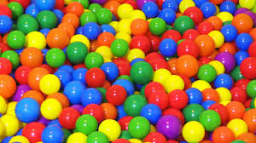Did you find an error or inaccuracy? Feel free to write us. Thank you!Tips to related online calculators
Check out our ratio calculator.
Do you want to perform natural numbers division - find the quotient and remainder?

## Related math problems and questions:

• Dividing moneyMilan divided 360 euros in the ratio 4: 5, Erik in the ratio 500: 625. How many euros did the individual parts of Milan and how many Erik?
• Three friendsThree friends had balls in ratio 2: 7: 4 at the start of the game. Could they have the same number of balls at the end of the game? Write 0, if not, or write the minimum number of balls they had together.
• Euros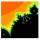Michal, Peter, John and Lenka got together 2,400 euros. They share an amount in ratio 2:6:4:3. How many got each of them?
• Birthdate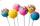Jane on birthday brought 30 lollipops and 24 chewing gum for their friends. How many friends has, if everyone receives the same number of lollipops and chewing gums? How much chewing gum and lollipops got any friend?
• AthletesAthletes at the stadium could enter two-steps, three-steps, four-steps, five-steps, six-steps. There were more than 100 but less than 200. How many athletes were there?
• DonutsFind how many donuts each student will receive if you share 126 donuts in a ratio of 1:5:8
• Sheep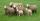Shepherd tending the sheep. Tourists asked him how much they have. The shepherd said, "there are fewer than 500. If I them lined up in 4-row 3 remain. If in 5-row 4 remain. If in 6-row 5 remain. But I can form 7-row." How many sheep have herdsman?
• Oranges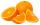Mother divided her three children's oranges in a ratio of 6:5:4. Two children gave 45 oranges. How many oranges were there?
• Twenty-fiveHow many are three-digit natural numbers divisible by 25?
• Divide 5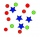Divide 288 in the following ratio 3 : 4 : 5
• GroupA group of kids wanted to ride. When the children were divided into groups of 3 children, one remain. When divided into groups of 4 children, 1 remains. When divided into groups of 6 children, 1 remains. After divided into groups of 5 children, no one lef
• 120 nuts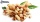Divide 120 nuts in a ratio of 4: 6.
• ErikThree friends Peter, Erik and Milan shared 420 euros in a ratio of 1: 2: 3. How many euros did Erik get?
• Dance groupThe dance group formed groups of 4, 5, and 6 members. Always one dancer remains. How many dancers were there in the whole group?
• Ducats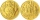The king divided the ducats to his three sons in a ratio of 2: 5: 4. How many ducats the king divided them if the youngest received 260 ducats, the least of all sons.
• HostelStudents are accommodated in 22 rooms. Rooms were 4 and 6 bed. How many rooms in which type occupied 106 children there?
• TogetherIf 8 men, 10 women, 16 children collects ₹1024 in 4 days, how many days will be required for 6 men, 5 women and 4 boys to collect ₹768? (₹ is Indian Rupee)